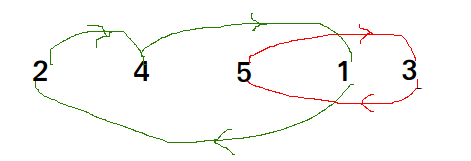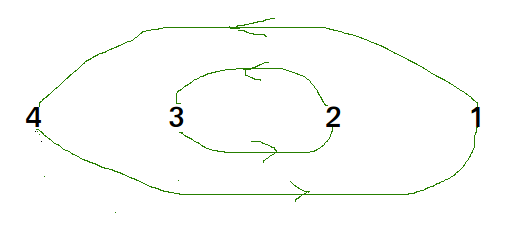Related Articles
Cycle Sort
• Difficulty Level : Medium
• Last Updated : 25 Mar, 2021

Cycle sort is an in-place sorting Algorithm, unstable sorting algorithm, a comparison sort that is theoretically optimal in terms of the total number of writes to the original array.

• It is optimal in terms of number of memory writes. It minimizes the number of memory writes to sort (Each value is either written zero times, if it’s already in its correct position, or written one time to its correct position.)
• It is based on the idea that array to be sorted can be divided into cycles. Cycles can be visualized as a graph. We have n nodes and an edge directed from node i to node j if the element at i-th index must be present at j-th index in the sorted array.
Cycle in arr[] = {2, 4, 5, 1, 3}• Cycle in arr[] = {4, 3, 2, 1}•

We one by one consider all cycles. We first consider the cycle that includes first element. We find correct position of first element, place it at its correct position, say j. We consider old value of arr[j] and find its correct position, we keep doing this till all elements of current cycle are placed at correct position, i.e., we don’t come back to cycle starting point.

Explanation :

``` arr[] = {10, 5, 2, 3}
index =  0   1   2   3
cycle_start = 0
item = 10 = arr

Find position where we put the item
pos = cycle_start
i=pos+1
while(i<n)
if (arr[i] < item)
pos++;

We put 10 at arr and change item to
old value of arr.
arr[] = {10, 5, 2, 10}
item = 3

Again rotate rest cycle that start with index '0'
Find position where we put the item = 3
we swap item with element at arr now
arr[] = {10, 3, 2, 10}
item = 5

Again rotate rest cycle that start with index '0' and item = 5
we swap item with element at arr.
arr[] = {10, 3, 5, 10 }
item = 2

Again rotate rest cycle that start with index '0' and item = 2
arr[] = {2, 3,  5, 10}

Above is one iteration for cycle_stat = 0.
Repeat above steps for cycle_start = 1, 2, ..n-2```

## CPP

 `// C++ program to implement cycle sort``#include ``using` `namespace` `std;` `// Function sort the array using Cycle sort``void` `cycleSort(``int` `arr[], ``int` `n)``{``    ``// count number of memory writes``    ``int` `writes = 0;` `    ``// traverse array elements and put it to on``    ``// the right place``    ``for` `(``int` `cycle_start = 0; cycle_start <= n - 2; cycle_start++) {``        ``// initialize item as starting point``        ``int` `item = arr[cycle_start];` `        ``// Find position where we put the item. We basically``        ``// count all smaller elements on right side of item.``        ``int` `pos = cycle_start;``        ``for` `(``int` `i = cycle_start + 1; i < n; i++)``            ``if` `(arr[i] < item)``                ``pos++;` `        ``// If item is already in correct position``        ``if` `(pos == cycle_start)``            ``continue``;` `        ``// ignore all duplicate  elements``        ``while` `(item == arr[pos])``            ``pos += 1;` `        ``// put the item to it's right position``        ``if` `(pos != cycle_start) {``            ``swap(item, arr[pos]);``            ``writes++;``        ``}` `        ``// Rotate rest of the cycle``        ``while` `(pos != cycle_start) {``            ``pos = cycle_start;` `            ``// Find position where we put the element``            ``for` `(``int` `i = cycle_start + 1; i < n; i++)``                ``if` `(arr[i] < item)``                    ``pos += 1;` `            ``// ignore all duplicate  elements``            ``while` `(item == arr[pos])``                ``pos += 1;` `            ``// put the item to it's right position``            ``if` `(item != arr[pos]) {``                ``swap(item, arr[pos]);``                ``writes++;``            ``}``        ``}``    ``}` `    ``// Number of memory writes or swaps``    ``// cout << writes << endl ;``}` `// Driver program to test above function``int` `main()``{``    ``int` `arr[] = { 1, 8, 3, 9, 10, 10, 2, 4 };``    ``int` `n = ``sizeof``(arr) / ``sizeof``(arr);``    ``cycleSort(arr, n);` `    ``cout << ``"After sort : "` `<< endl;``    ``for` `(``int` `i = 0; i < n; i++)``        ``cout << arr[i] << ``" "``;``    ``return` `0;``}`

## Java

 `// Java program to implement cycle sort` `import` `java.util.*;``import` `java.lang.*;` `class` `GFG {``    ``// Function sort the array using Cycle sort``    ``public` `static` `void` `cycleSort(``int` `arr[], ``int` `n)``    ``{``        ``// count number of memory writes``        ``int` `writes = ``0``;` `        ``// traverse array elements and put it to on``        ``// the right place``        ``for` `(``int` `cycle_start = ``0``; cycle_start <= n - ``2``; cycle_start++) {``            ``// initialize item as starting point``            ``int` `item = arr[cycle_start];` `            ``// Find position where we put the item. We basically``            ``// count all smaller elements on right side of item.``            ``int` `pos = cycle_start;``            ``for` `(``int` `i = cycle_start + ``1``; i < n; i++)``                ``if` `(arr[i] < item)``                    ``pos++;` `            ``// If item is already in correct position``            ``if` `(pos == cycle_start)``                ``continue``;` `            ``// ignore all duplicate elements``            ``while` `(item == arr[pos])``                ``pos += ``1``;` `            ``// put the item to it's right position``            ``if` `(pos != cycle_start) {``                ``int` `temp = item;``                ``item = arr[pos];``                ``arr[pos] = temp;``                ``writes++;``            ``}` `            ``// Rotate rest of the cycle``            ``while` `(pos != cycle_start) {``                ``pos = cycle_start;` `                ``// Find position where we put the element``                ``for` `(``int` `i = cycle_start + ``1``; i < n; i++)``                    ``if` `(arr[i] < item)``                        ``pos += ``1``;` `                ``// ignore all duplicate elements``                ``while` `(item == arr[pos])``                    ``pos += ``1``;` `                ``// put the item to it's right position``                ``if` `(item != arr[pos]) {``                    ``int` `temp = item;``                    ``item = arr[pos];``                    ``arr[pos] = temp;``                    ``writes++;``                ``}``            ``}``        ``}``    ``}` `    ``// Driver program to test above function``    ``public` `static` `void` `main(String[] args)``    ``{``        ``int` `arr[] = { ``1``, ``8``, ``3``, ``9``, ``10``, ``10``, ``2``, ``4` `};``        ``int` `n = arr.length;``        ``cycleSort(arr, n);` `        ``System.out.println(``"After sort : "``);``        ``for` `(``int` `i = ``0``; i < n; i++)``            ``System.out.print(arr[i] + ``" "``);``    ``}``}` `// Code Contributed by Mohit Gupta_OMG <(0_o)>`

## Python3

 `# Python program to implement cycle sort` `def` `cycleSort(array):``  ``writes ``=` `0``  ` `  ``# Loop through the array to find cycles to rotate.``  ``for` `cycleStart ``in` `range``(``0``, ``len``(array) ``-` `1``):``    ``item ``=` `array[cycleStart]``    ` `    ``# Find where to put the item.``    ``pos ``=` `cycleStart``    ``for` `i ``in` `range``(cycleStart ``+` `1``, ``len``(array)):``      ``if` `array[i] < item:``        ``pos ``+``=` `1``    ` `    ``# If the item is already there, this is not a cycle.``    ``if` `pos ``=``=` `cycleStart:``      ``continue``    ` `    ``# Otherwise, put the item there or right after any duplicates.``    ``while` `item ``=``=` `array[pos]:``      ``pos ``+``=` `1``    ``array[pos], item ``=` `item, array[pos]``    ``writes ``+``=` `1``    ` `    ``# Rotate the rest of the cycle.``    ``while` `pos !``=` `cycleStart:``      ` `      ``# Find where to put the item.``      ``pos ``=` `cycleStart``      ``for` `i ``in` `range``(cycleStart ``+` `1``, ``len``(array)):``        ``if` `array[i] < item:``          ``pos ``+``=` `1``      ` `      ``# Put the item there or right after any duplicates.``      ``while` `item ``=``=` `array[pos]:``        ``pos ``+``=` `1``      ``array[pos], item ``=` `item, array[pos]``      ``writes ``+``=` `1``  ` `  ``return` `writes``  ` `# driver code``arr ``=` `[``1``, ``8``, ``3``, ``9``, ``10``, ``10``, ``2``, ``4` `]``n ``=` `len``(arr)``cycleSort(arr)` `print``(``"After sort : "``)``for` `i ``in` `range``(``0``, n) :``    ``print``(arr[i], end ``=` `' '``)` `# Code Contributed by Mohit Gupta_OMG <(0_o)>`

## C#

 `// C# program to implement cycle sort``using` `System;` `class` `GFG {``    ` `    ``// Function sort the array using Cycle sort``    ``public` `static` `void` `cycleSort(``int``[] arr, ``int` `n)``    ``{``        ``// count number of memory writes``        ``int` `writes = 0;` `        ``// traverse array elements and``        ``// put it to on the right place``        ``for` `(``int` `cycle_start = 0; cycle_start <= n - 2; cycle_start++)``        ``{``            ``// initialize item as starting point``            ``int` `item = arr[cycle_start];` `            ``// Find position where we put the item.``            ``// We basically count all smaller elements``            ``// on right side of item.``            ``int` `pos = cycle_start;``            ``for` `(``int` `i = cycle_start + 1; i < n; i++)``                ``if` `(arr[i] < item)``                    ``pos++;` `            ``// If item is already in correct position``            ``if` `(pos == cycle_start)``                ``continue``;` `            ``// ignore all duplicate elements``            ``while` `(item == arr[pos])``                ``pos += 1;` `            ``// put the item to it's right position``            ``if` `(pos != cycle_start) {``                ``int` `temp = item;``                ``item = arr[pos];``                ``arr[pos] = temp;``                ``writes++;``            ``}` `            ``// Rotate rest of the cycle``            ``while` `(pos != cycle_start) {``                ``pos = cycle_start;` `                ``// Find position where we put the element``                ``for` `(``int` `i = cycle_start + 1; i < n; i++)``                    ``if` `(arr[i] < item)``                        ``pos += 1;` `                ``// ignore all duplicate elements``                ``while` `(item == arr[pos])``                    ``pos += 1;` `                ``// put the item to it's right position``                ``if` `(item != arr[pos]) {``                    ``int` `temp = item;``                    ``item = arr[pos];``                    ``arr[pos] = temp;``                    ``writes++;``                ``}``            ``}``        ``}``    ``}` `    ``// Driver program to test above function``    ``public` `static` `void` `Main()``    ``{``        ``int``[] arr = { 1, 8, 3, 9, 10, 10, 2, 4 };``        ``int` `n = arr.Length;``        ` `        ``// Function calling``        ``cycleSort(arr, n);` `        ``Console.Write(``"After sort : "``);``        ``for` `(``int` `i = 0; i < n; i++)``            ``Console.Write(arr[i] + ``" "``);``    ``}``}` `// This code is contributed by Nitin Mittal`

## Javascript

 ``

Output:

```After sort :
1 2 3 4 8 9 10 10 ```

Time Complexity : O(n2
Worst Case : O(n2
Average Case: O(n2
Best Case : O(n2)
This sorting algorithm is best suited for situations where memory write or swap operations are costly.

Reference:
https://en.wikipedia.org/wiki/Cycle_sort
This article is contributed by Nishant Singh. If you like GeeksforGeeks and would like to contribute, you can also write an article using contribute.geeksforgeeks.org or mail your article to contribute@geeksforgeeks.org. See your article appearing on the GeeksforGeeks main page and help other Geeks.
Please write comments if you find anything incorrect, or you want to share more information about the topic discussed above.

Attention reader! Don’t stop learning now. Get hold of all the important DSA concepts with the DSA Self Paced Course at a student-friendly price and become industry ready.

My Personal Notes arrow_drop_up